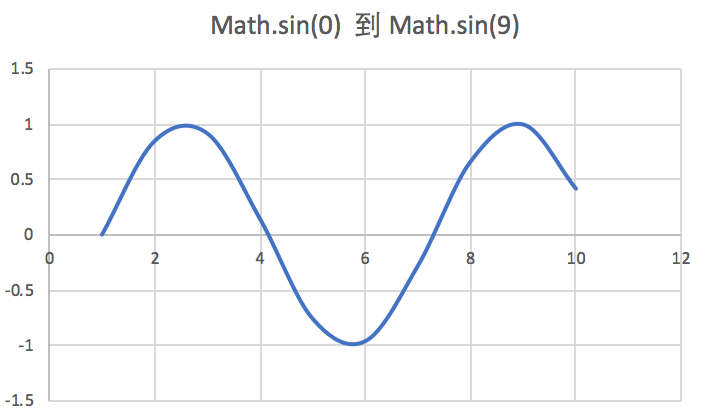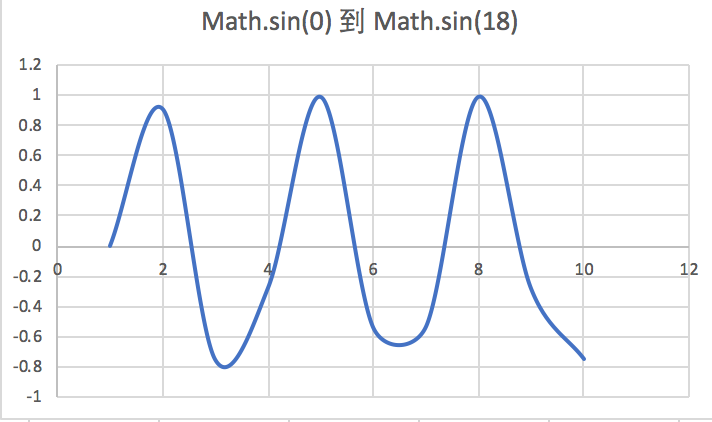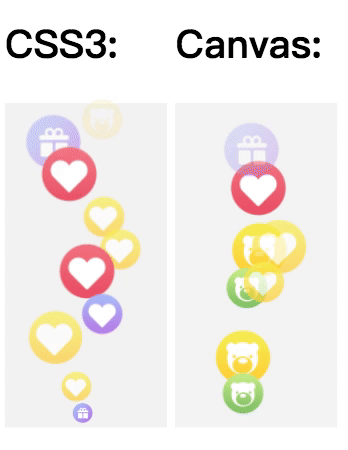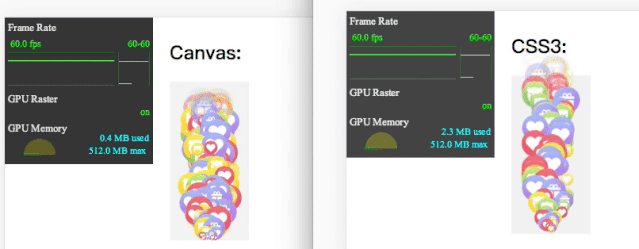# H5 直播的疯狂点赞动画是如何实现的？

• 点赞动作无限次，引导用户疯狂点赞
• 直播间的所有疯狂点赞，都需要在所有用户界面都动画展现出来(广播用户使用websocket消息)• 点赞动画图片大小不一，运动轨迹也是随机的
• 点赞动画图片都是先放大再匀速运动。
• 快到顶部的时候，是渐渐消失。
• 收到大量的点赞请求的时候，点赞动画不扎堆，井然有序持续出现。

## CSS3 实现

``````animation: name duration timing-function delay iteration-count direction fill-mode play-state;
``````

### Step 1: 固定区域，设置基本样式``````<div class="praise_bubble">
<div class="bubble b1 bl1"></div>
</div>
``````
``````.praise_bubble{
width:100px;
height:200px;
position:relative;
background-color:#f4f4f4;
}
.bubble{
position: absolute;
left:50%;
bottom:0;
}
``````### Step 2: 运动起来

``````.bl1{
animation:bubble_y 4s linear 1 forwards ;
}
@keyframes bubble_y {
0% {
margin-bottom:0;
}
100% {
margin-bottom:200px;
}
}
``````### Step 3: 增加渐隐

``````@keyframes bubble_y {
0% {
margin-bottom:0;
}
75%{
opacity:1;
}
100% {
margin-bottom:200px;
opacity:0;
}
}
``````### Step 4: 增加动画放大效果

``````.bl1{
animation:bubble_big 0.5s linear 1 forwards;
}
@keyframes bubble_big_1 {
0% {
transform: scale(.3);
}
100% {
transform: scale(1);
}
}
``````### Step 5: 设置偏移

``````@keyframes bubble_1 {
0% {
}
25% {
margin-left:-8px;
}
50% {
margin-left:8px
}
75% {
margin-left:-15px
}
100% {
margin-left:15px
}
}
``````### Step 7: JS 操作随机增加节点样式

``````let praiseBubble = document.getElementById("praise_bubble");
let last = 0;
const b =Math.floor(Math.random() * 6) + 1;
const bl =Math.floor(Math.random() * 11) + 1; // bl1~bl11
let d = document.createElement("div");
d.className = `bubble b\${b} bl\${bl}`;
d.dataset.t = String(Date.now());
praiseBubble.appendChild(d);
}
setInterval(() => {
},300)
``````

``````.bl2{
animation:bubble_2 \$bubble_time linear .4s 1 forwards,bubble_big_2 \$bubble_scale linear .4s 1 forwards,bubble_y \$bubble_time linear .4s 1 forwards;
}
``````

`````` window.requestAnimationFrame(() => {
// 继续循环处理批次
render();
});
``````## Canvas 绘图实现

### Step 1:初始化

canvas 上可以设置 width 和 height 属性，也可以在 style 属性里面设置 width 和 height。

• canvas 上 style 的 width 和 height 是 canvas 在浏览器中被渲染的高度和宽度，即在页面中的实际宽高。
• canvas 标签的 width 和 height 是画布实际宽度和高度。
``````<canvas id="thumsCanvas" width="200" height="400" style="width:100px;height:200px"></canvas>
``````

``````class ThumbsUpAni{
constructor(){
const canvas = document.getElementById('thumsCanvas');
this.context = canvas.getContext('2d')!;
this.width = canvas.width;
this.height = canvas.height;
}
}
``````

### Step 2：提前加载图片资源

``````loadImages(){
const images = [
'jfs/t1/93992/8/9049/4680/5e0aea04Ec9dd2be8/608efd890fd61486.png',
'jfs/t1/108305/14/2849/4908/5e0aea04Efb54912c/bfa59f27e654e29c.png',
'jfs/t1/94291/26/9105/4344/5e0aea05Ed64b9187/5165fdf5621d5bbf.png',
'jfs/t1/102753/34/8504/5522/5e0aea05E0b9ef0b4/74a73178e31bd021.png',
'jfs/t1/102954/26/9241/5069/5e0aea05E7dde8bda/720fcec8bc5be9d4.png'
];
const promiseAll = [] as Array<Promise<any>>;
images.forEach((src) => {
const p = new Promise(function (resolve) {
const img = new Image;
img.onerror = img.onload = resolve.bind(null, img);
});
promiseAll.push(p);
});
Promise.all(promiseAll).then((imgsList) => {
this.imgsList = imgsList.filter((d) => {
if (d && d.width > 0) return true;
return false;
});
if (this.imgsList.length == 0) {
return;
}
})
}
``````

### 随机平滑 X 轴偏移``````const angle = getRandom(2, 10);
let ratio = getRandom(10,30)*((getRandom(0, 1) ? 1 : -1));
const getTranslateX = (diffTime) => {
if (diffTime < this.scaleTime) {// 放大期间，不进行摇摆偏移
return basicX;
} else {
return basicX + ratio*Math.sin(angle*(diffTime - this.scaleTime));
}
};
``````

scaleTime 是从开始放大到最终大小，用多长时间，这里我们设置 0.1，即总共运行时间前面的 10% 的时间，点赞图片逐步放大。

diffTime，是只从开始动画运行到当前时间过了多长时间了，为百分比。实际值是从 0  --》 1 逐步增大。diffTime - scaleTime = 0 ~ 0.9, diffTime 为 0.4 的时候，说明是已经运行了 40% 的时间。### Y 轴偏移

``````const getTranslateY = (diffTime) => {
return image.height / 2 + (this.height - image.height / 2) * (1-diffTime);
};
``````

### 放大缩小

``````const basicScale = [0.6, 0.9, 1.2][getRandom(0, 2)];
const getScale = (diffTime) => {
if (diffTime < this.scaleTime) {
return +((diffTime/ this.scaleTime).toFixed(2)) * basicScale;
} else {
return basicScale;
}
};
``````

### 淡出

``````const fadeOutStage = getRandom(14, 18) / 100;
const getAlpha = (diffTime) => {
let left = 1 - +diffTime;
return 1;
} else {
}
};
``````

## 实时绘制

``````createRender(){
return (diffTime) => {
// 差值满了，即结束了 0 ---》 1
if(diffTime>=1) return true;
context.save();
const scale = getScale(diffTime);
const translateX = getTranslateX(diffTime);
const translateY = getTranslateY(diffTime);
context.translate(translateX, translateY);
context.scale(scale, scale);
context.globalAlpha = getAlpha(diffTime);
// const rotate = getRotate();
// context.rotate(rotate * Math.PI / 180);
context.drawImage(
image,
-image.width / 2,
-image.height / 2,
image.width,
image.height
);
context.restore();
};
}
``````

``````const basicScale = [0.6, 0.9, 1.2][getRandom(0, 2)];
``````

## 实时绘制扫描器

``````scan() {
this.context.clearRect(0, 0, this.width, this.height);
this.context.fillStyle = "#f4f4f4";
this.context.fillRect(0,0,200,400);
let index = 0;
let length = this.renderList.length;
if (length > 0) {
requestAnimationFrame(this.scan.bind(this));
}
while (index < length) {
const render = this.renderList[index];
if (!render || !render.render || render.render.call(null, (Date.now() - render.timestamp) / render.duration)) {
// 结束了，删除该动画
this.renderList.splice(index, 1);
length--;
} else {
// 当前动画未执行完成，continue
index++;
}
}
}
``````

``````diffTime = (Date.now() - render.timestamp) / render.duration
``````

## 增加动画

``````start() {
const render = this.createRender();
const duration = getRandom(1500, 3000);
this.renderList.push({
render,
duration,
timestamp: Date.now(),
});
if (!this.scanning) {
this.scanning = true;
requestFrame(this.scan.bind(this));
}
return this;
}
``````

### 保持不扎堆

``````thumbsUp(num: number) {
if (num <= this.praiseLast) return;
this.thumbsStart = this.praiseLast;
this.praiseLast = num;
if (this.thumbsStart + 500 < num)
this.thumbsStart = num - 500;
const diff = this.praiseLast - this.thumbsStart;
let time = 100;
let isFirst = true;
if (this.thumbsInter != 0) {
return;
}
this.thumbsInter = setInterval(() => {
if (this.thumbsStart >= this.praiseLast) {
clearInterval(this.thumbsInter);
this.thumbsInter = 0;
return;
}
this.thumbsStart++;
this.thumbsUpAni.start();
if (isFirst) {
isFirst = false;
time = Math.round(5000 / diff);
}
}, time);
},
``````

• 对于热门直播，会同时渲染的动画很多，不会扎堆显示，且动画完全能衔接上，不停的冒泡点赞动画。
• 对于冷门直播，有多余一个的点赞请求，我们能打散到 5s 内显示，也不会扎堆显示。

## End## 再比较• CSS3 实现简单
• Canvas 更灵活，操作更细腻
• CSS3 内存消耗比 Canvas 大，如果开启硬件加速，内存消耗更大一些。